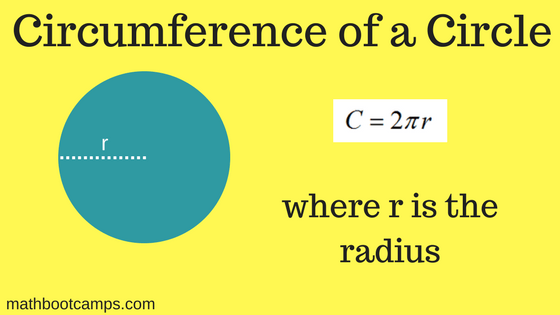# The circumference of a circle

The circumference of a circle is the distance around the outside of a circle, which can be calculated using the radius $$r$$ and the circumference formula $$C = 2\pi r$$. Since it is a distance, the answer is given using any unit of length such as feet, inches, meters, or kilometers. In the lesson below, we will look at the different types of problems that use the circumference, including word problems.

## Examples of finding the circumference of a circle

First, we will consider some mathematical examples where you are given the radius or the diameter and simply asked to find the circumference. As with area questions, it is important to pay attention to which value you are given since this affects the calculation (remember the diameter is always twice the radius).Let’s do an example of each of the two cases and see how the formula is applied in the different situations.

The radius of a circle is 3 cm. What is the circumference of this circle?

### Solution

Apply the circumference formula with $$r = 3$$.

\begin{align}C &= 2\pi r\\ &= 2\pi(3)\\ &= \bbox[border: 1px solid black; padding: 2px]{6\pi \text{ cm}}\end{align}

You could also use $$\pi = 3.14$$ and approximate the answer as $$6 \times 3.14 = 18.84 \text{ cm}$$.

### Example (given diameter)

A circle has a diameter of 10 feet. What is the circle’s circumference?

### Solution

Remember that the radius of a circle is half the diameter. Therefore, you will use $$r = 5\text{ feet}$$ in the circumference formula.

\begin{align}C &= 2\pi r\\ &= 2\pi(5) \\ &= \bbox[border: 1px solid black; padding: 2px]{10\pi \text{ feet}}\end{align}

As before, you could approximate this as $$10 \times 3.14 = 31.4 \text{ feet}$$.

These examples were the types you will see when you are first learning how to use this formula. But it is also possible to solve applied and other types of word problems using the circumference formula. We will look at those next.

## Word problems using the circumference

To solve any type of applied problem in geometry, you must be able to identify the values you need to find and those you are given. This is based on your understanding of geometry ideas like area, perimeter, and volume – or really any idea – depending on the problem.

When it comes to circumference, that means understanding that it represents the distance around the circle. So, if you are given a real-life situation involving finding a distance like this you know to apply the circumference formula.

### Example

A group has raised money to build a memorial fountain in the center of town. The fountain will be circular with a small statue in the center. The distance from the center of the statue to the outer edge of the fountain will be 15 feet. On the day the fountain is complete, the group will place a ribbon around the outside of the circle to be cut in a ceremony. How many feet of ribbon will they need? Assume that the ribbon is placed 6 inches outside of the edge of the fountain, all the way around.

### Solution

If the statue is at the center of the circle, then the distance from the statue to the outside of the fountain is the radius. But the ribbon will enclose a circle that has a radius 6 inches, or half a foot, larger than this. Therefore we should use:

$$r = 15.5 \text{ feet}$$

Now we can apply the formula to find:

\begin{align}C &= 2\pi r \\&= 2\pi(15.5)\\ &= 31\pi\\ &\approx 31 \times 3.14 \\&= \bbox[border: 1px solid black; padding: 2px]{97.3 \text{ feet}}\end{align}

Now while this example used a real-life situation, some word problems are a more mathematical in nature and may require you to use your knowledge of algebra. This is true in the next example, where you are given the circumference, but must find the diameter of the circle.

### Example

The circumference of a circle is 3 meters. In terms of $$\pi$$, what is the diameter of this circle?

### Solution

With the information given, we know that:

$$C = 3 \text{ meters} = 2\pi r$$

We can solve for $$r$$ by dividing both sides by $$2 \pi$$.

$$\dfrac{3}{2\pi} = r$$

However, the question asks us to find the diameter, $$d$$. This is twice the radius. Therefore:

\begin{align}d &= 2r\\ &= 2 \times \dfrac{3}{2\pi}\\ &= \bbox[border: 1px solid black; padding: 2px]{\dfrac{3}{\pi} \text{ meters}}\end{align}

You wouldn’t round here since the question asked for the value “in terms of $$\pi$$;”.

## Summary

Circumference is really just another way of talking about the perimeter, or the distance around, a circle. This can be calculated in exact form (in terms of $$\pi$$ or as a decimal approximation using the circumference formula. From there you can also solve applied problems or more complicated mathematical problems using your general understanding of circles and their properties.

## Continue studying circles

You can review how to find the area of a circle in the following lesson: Finding the area of a circle